# The Many Faces of Map

Episode #13 • Apr 23, 2018 • Subscriber-Only

Why does the `map` function appear in every programming language supporting “functional” concepts? And why does Swift have two `map` functions? We will answer these questions and show that `map` has many universal properties, and is in some sense unique.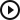The Many Faces of Map
Introduction
00:05
Swift’s maps
01:08
07:59
17:02
The f word
27:20
What’s the point?
29:40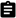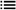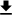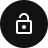### Introduction

Today’s topic is a big one, and it’s only the beginning of a long, deep journey. We want to consider the seemingly humble `map` function in all of its glory. Many people point to the presence of `map` in a language as being a strong indicator of the language having “functional” tendencies. You’ll often hear “language ABC has support for functional concepts like `map`, `filter`, `reduce`, etc”, and the mention of `map` is always first! Why is that?!

Swift must be doubly functional because it comes with two `map`s! One on arrays and one on optionals!

We want to build intuition for why `map` seems to be so ubiquitous in “functional-leaning” languages, and in fact there are lots of other `map`s lurking in the shadows of our everyday Swift code that we have yet to explore.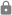This episode is for subscribers only.

### Subscribe to Point-Free

Access this episode, plus all past and future episodes when you become a subscriber.

See plans and pricing

### Exercises

1. Implement a `map` function on dictionary values, i.e.

``````map: ((V) -> W) -> ([K: V]) -> [K: W]
``````

Does it satisfy `map(id) == id`?

2. Implement the following function:

``````transformSet: ((A) -> B) -> (Set<A>) -> Set<B>
``````

We do not call this `map` because it turns out to not satisfy the properties of `map` that we saw in this episode. What is it about the `Set` type that makes it subtly different from `Array`, and how does that affect the genericity of the `map` function?

3. Recall that one of the most useful properties of `map` is the fact that it distributes over compositions, i.e. `map(f >>> g) == map(f) >>> map(g)` for any functions `f` and `g`. Using the `transformSet` function you defined in a previous example, find an example of functions `f` and `g` such that

``````transformSet(f >>> g) != transformSet(f) >>> transformSet(g)
``````

This is why we do not call this function `map`.

4. There is another way of modeling sets that is different from `Set<A>` in the Swift standard library. It can also be defined as function `(A) -> Bool` that answers the question “is `a: A` contained in the set.” Define a type `struct PredicateSet<A>` that wraps this function. Can you define the following?

``````map: ((A) -> B) -> (PredicateSet<A>) -> PredicateSet<B>
``````

What goes wrong?

5. Try flipping the direction of the arrow in the previous exercise. Can you define the following function?

``````fakeMap: ((B) -> A) -> (PredicateSet<A>) -> PredicateSet<B>
``````
6. What kind of laws do you think `fakeMap` should satisfy?

7. Sometimes we deal with types that have multiple type parameters, like `Either` and `Result`. For those types you can have multiple `map`s, one for each generic, and no one version is “more” correct than the other. Instead, you can define a `bimap` function that takes care of transforming both type parameters at once. Do this for `Result` and `Either`.

8. Write a few implementations of the following function:

``````func r<A>(_ xs: [A]) -> A? {
}
``````
9. Continuing the previous exercise, can you generalize your implementations of `r` to a function `[A] -> B?` if you had a function `f: (A) -> B`?

``````func s<A, B>(_ f: (A) -> B, _ xs: [A]) -> B? {
}
``````

What features of arrays and optionals do you need to implement this?

10. Derive a relationship between `r`, any function `f: (A) -> B`, and the `map` on arrays and optionals.

This relationship is the “free theorem” for `r`’s signature.